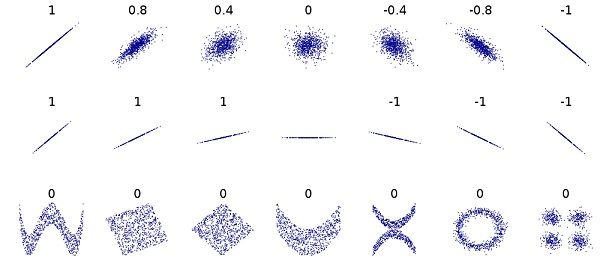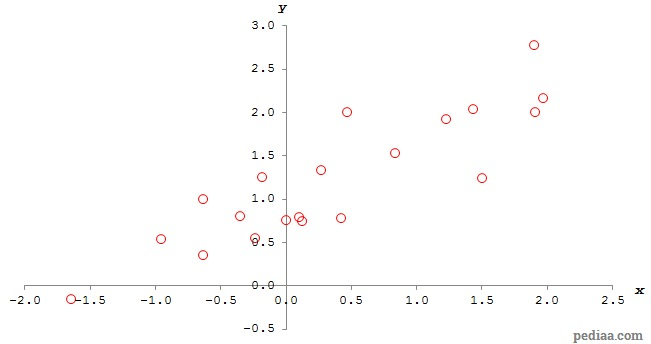# Difference Between Correlation and Regression

## Main Difference – Correlation vs. Regression

Correlation and regression are two methods used to investigate the relationship between variables in statistics. The main difference between correlation and regression is that correlation measures the degree to which the two variables are related, whereas regression is a method for describing the relationship between two variables. Regression also allows one to more accurately predict the value that the dependent variable would take for a given value of the independent variable.

## What is Correlation

In statistics, we say there is a correlation between two variables if the two variables are related. If the relationship between the variables is a linear one, we can express the degree to which they are related using a number called Pearson’s correlation coefficient$\mathbf{\left( \mathit {\rho}\right)}$$\rho$ takes a value between -1 and 1. A value of 0 means that the two variables are uncorrelated. Negative values indicate that the correlation between the variables is negative: i.e. as one variable increases, the other variable decreases. Similarly, a positive value for$\rho$ means that the data is positively correlated (when one variable increases, the other variable increases too).

A value of$\rho$ that is -1 or 1 gives the strongest possible correlation. When$\rho =-1$ the variables are said to be completely negatively correlated and when$\rho =1$ the values are said to be completely positively correlated. The figure below shows several shapes of scatter plots between two variables and the correlation coefficient for each case:Pearson’s correlation coefficient for different types of scatter plots

Pearson’s correlation coefficient for two variables$x$ and$y$ is defined as follows:$\rho=\frac{\mathrm{cov\left( \mathit{x,y}\right )}}{\sigma_{x}\sigma_{y}}$

Here,$\mathrm{cov}\left( \mathit{x,y}\right )$ is the covariance between$x$ and$y$:$\mathrm{cov}\left( \mathit{x,y}\right )=\frac{1}{N}\sum_{i=1}^{N}\left( x_i-\bar{x}\right)\left( y_i-\bar{y}\right)=\left( \frac{1}{N}\sum_{i=1}^{N} x_iy_i\right)-\bar{x}\bar{y}$

The terms$\sigma_x$ and$\sigma_y$ stand for standard deviations of$x$ and$y$ respectively.This is defined as:$\sigma_x=\sqrt{\frac{1}{N}\sum_{i=1}^{N}{\left( x_i-\bar{x}\right )}^2}$ and$\sigma_y=\sqrt{\frac{1}{N}\sum_{i=1}^{N}{\left( y_i-\bar{y}\right )}^2}$

Let us see how the correlation coefficient is calculated using an example. We will try to calculate the correlation coefficient for the following set of 20 values for$x$ and$y$:$x$$y$ -0.9557 0.5369 -1.6441 -0.1560 1.2254 1.9230 1.9062 1.9957 1.9679 2.1673 -0.3469 0.7954 -0.2328 0.5415 1.5064 1.2335 0.4278 0.7754 -0.6359 0.3534 0.0061 0.7565 0.8407 1.5326 0.2713 1.3354 0.4664 1.9980 -0.1813 1.2539 1.4384 2.0383 1.9001 2.7755 0.1022 0.7861 0.1251 0.7456 -0.6314 0.9942

The values of$y$ are plotted against the values of$x$ on the graph shown below:Looking at the equations needed to calculate the correlation coefficient, we will first calculate values for$\bar{x}, \bar{y}$. These are the mean values of$x$ and$y$ respectively. We find that:$\bar{x}=0.3778$$\bar{y}=1.2191$

Next, we will calculate$x_iy_i, {\left( x_i-\bar{x}\right )}^2,$ and${\left( y_i-\bar{y}\right )}^2$. We will put these values next to our values of$x$ and$y$ on the table above:$x$$y$$x_iy_i$${\left( x_i-\bar{x}\right )}^2$${\left( y_i-\bar{y}\right )}^2$ -0.9557 0.5369 -0.5131 1.7782 0.4654 -1.6441 -0.1560 0.2565 4.0881 1.8909 1.2254 1.9230 2.3564 0.7184 0.4955 1.9062 1.9957 3.8042 2.3360 0.6031 1.9679 2.1673 4.2650 2.5284 0.8991 -0.3469 0.7954 -0.2759 0.5252 0.1795 -0.2328 0.5415 -0.1261 0.3728 0.4592 1.5064 1.2335 1.8581 1.2737 0.0002 0.4278 0.7754 0.3317 0.0025 0.1969 -0.6359 0.3534 -0.2247 1.0276 0.7495 0.0061 0.7565 0.0046 0.1382 0.2140 0.8407 1.5326 1.2885 0.2143 0.0983 0.2713 1.3354 0.3623 0.0113 0.0135 0.4664 1.9980 0.9319 0.0079 0.6067 -0.1813 1.2539 -0.2273 0.3126 0.0012 1.4384 2.0383 2.9319 1.1249 0.6711 1.9001 2.7755 5.2737 2.3174 2.4223 0.1022 0.7861 0.0803 0.0760 0.1875 0.1251 0.7456 0.0933 0.0639 0.2242 -0.6314 0.9942 -0.6277 1.0185 0.0506

With these values, we can calculate the covariance:$\frac{1}{N}\sum_{i=1}^{N} x_iy_i=1.0922$$\bar{x}\bar{y}=0.4606$$\therefore \mathrm{cov}\left( \mathit{x,y}\right)=1.0922-0.4606=0.6316$

We can also calculate the standard deviations:$\sum_{i=1}^{N}{\left( x_i-\bar{x}\right )}^2=19.94$$\sigma_x=\sqrt{\frac{19.94}{20}}=0.9985$$\sum_{i=1}^{N}{\left( y_i-\bar{y}\right )}^2=10.43$$\sigma_y=\sqrt{\frac{10.43}{20}}=0.7221$$\sigma_x\sigma_y=0.7211$

Now we can calculate the correlation coefficient:$\rho=\frac{\mathrm{cov\left( \mathit{x,y}\right )}}{\sigma_{x}\sigma_{y}}=\frac{0.6316}{0.7221}=0.876$

## What is Regression

Regression is a method for finding the relationship between two variables. Specifically, we will look at linear regression, which gives an equation for a “line of best fit” for a given sample of data, where two variables have a linear relationship. A straight line can be described with an equation in the form of$y=mx+c$ where$m$ is the gradient of the line and$c$ axis, and linear regression allows us to calculate the values of$m$ and$c$. Once we have calculated the correlation coefficient$\rho$, we can calculate these values as:$m=\rho\left( \frac{\sigma_y}{\sigma_x}\right)$$c=\bar{y}-m\bar{x}$

Note that in these cases,$y$ is taken to be the dependent variable while$x$ is the independent variable. From our previous calculations, we know that$\rho=0.876$,$\sigma_x=0.9985$ and$\sigma_y=0.7221$. Therefore,$m=0.876\times\left( \frac{0.7221}{0.9985}\right)=0.634$.$\bar{y}=1.2191$ and$\bar{x}=0.3778$. Therefore,$c=1.2191-\left( 0.634\times 0.3778\right)=0.980$.

The image below shows the previous scatter plot with the line$y=0.634x+0.980$:

As we mentioned before, regression analysis aids us to make predictions. For instance, if the value of the independent variable ($x$) was 1.000, then we can predict that$y$ would be close to$y=\left( 0.634\times 1.000\right)+0.980=1.614$. In reality, the value of$y$ may not necessarily be exactly 1.614. Due to uncertainty, the actual value is likely to be different. Note that the accuracy of the prediction is higher for data with a correlation coefficient closer to ±1.

## Difference Between Correlation and Regression

### Describing Relationships

Correlation describes the degree to which two variables are related.

Regression gives a method for finding the relationship between two variables.

### Making Predictions

Correlation merely describes how well two variables are related. Analysing the correlation between two variables does not improve the accuracy with which the value of the dependent variable could be predicted for a given value of the independent variable.

Regression allows us to predict values of the dependent variable for a given value of the independent variable more accurately.

### Dependence Between Variables

In analysing correlation, it does not matter which variable is independent and which is independent.

In analysing regression, it is necessary to identify between the dependent and the independent variable.

Image Courtesy:

“redesign File:Correlation_examples.png using vector graphics (SVG file)” by DenisBoigelot (Own work, original uploader was Imagecreator) [CC0 1.0], via Wikimedia Commons